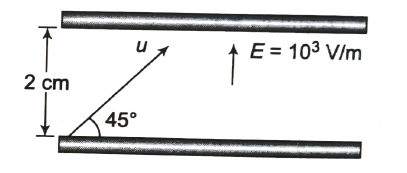A particle having charge that of an electron and mass 1.6x 10-30 kg is projected with an initial speed u at an angle 45° to the horizontal from the lower plate of a parallel plate capacitor as shown in figure. The plates are sufficiently long and have separation 2 cm. The maximum value of velocity of particle not to hit the upper plate is W×106 m/s. Take electric field between the plates = 103 V/m directed upward. The value of W is________

# A particle having charge that of an electron and mass 1.6x 10-30 kg is projected with an initial speed u at an angle 45° to the horizontal from the lower plate of a parallel plate capacitor as shown in figure. The plates are sufficiently long and have separation 2 cm. The maximum value of velocity of particle not to hit the upper plate is $\sqrt{W}×{10}^{6}$ m/s. Take electric field between the plates = 103 V/m directed upward. The value of W is________Register to Get Free Mock Test and Study Material

+91

Verify OTP Code (required)

### Solution:

Resolving the velocity of particle parallel and perpendicular to the plate.

Force on the charged particle in downward direction normal to the plate = eE
$\therefore$ Acceleration $a=\frac{eE}{m}$, where m is the mass of charged particle.
The particle will not hit the upper plate, if the velocity component normal to plate becomes zero before reaching if, i.e.,
$0={u}_{\perp }^{2}-2ay$, with $y\le d$ where d, distance between the plates.

$\therefore$ Maximum velocity for the particle not to hit the upper plate (for this y= d=2 cm)

$\begin{array}{l}{u}_{\perp }=\sqrt{2ay}=\sqrt{\frac{2×1.6×{10}^{-19}×{10}^{3}×2×{10}^{-2}}{1.6×{10}^{-30}}}=2×{10}^{6}\mathrm{m}/\mathrm{s}\\ {u}_{max}={u}_{\perp }/\mathrm{cos}{45}^{\circ }=2\sqrt{2}×{10}^{6}\mathrm{m}/\mathrm{s}=\sqrt{8}×{10}^{6}\mathrm{m}/\mathrm{s}\end{array}$Register to Get Free Mock Test and Study Material

+91

Verify OTP Code (required)